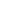Multiplication Times Tables Worksheets

Worksheets of times tables (multiplication 1 to 12)

Visual Multiplication Worksheets

Worksheets of visual multiplication

Double Digit Subtraction Worksheets (With Borrowing)

Worksheets of double digit subtraction (with borrowing)

Double Digit Subtraction Worksheets (No Borrowing)

Worksheets of double digit subtraction (no borrowing)

Single Digit Simple Subtraction Worksheets

Worksheets of single digit subtraction

Visual Subtraction Worksheets

Worksheets of visual subtraction

Picture Subtraction Worksheets

Worksheets of subtracting using pictures

Double Digit Addition Worksheets (with Carrying)

Worksheets of double digit addition (with carrying)

Double Digit Addition Worksheets (with No Carrying)

Worksheets of double digit addition (with no carrying)